Courses

# Test: Flywheels - 2

## 10 Questions MCQ Test Topicwise Question Bank for Mechanical Engineering | Test: Flywheels - 2

Description
This mock test of Test: Flywheels - 2 for Mechanical Engineering helps you for every Mechanical Engineering entrance exam. This contains 10 Multiple Choice Questions for Mechanical Engineering Test: Flywheels - 2 (mcq) to study with solutions a complete question bank. The solved questions answers in this Test: Flywheels - 2 quiz give you a good mix of easy questions and tough questions. Mechanical Engineering students definitely take this Test: Flywheels - 2 exercise for a better result in the exam. You can find other Test: Flywheels - 2 extra questions, long questions & short questions for Mechanical Engineering on EduRev as well by searching above.
QUESTION: 1

### In the case of a flywheel of mass moment of inertia 'I’ rotating at an angular velocity ‘ω’ the expression 1/2 lω2 represents the

Solution:

Kinetic energy stored in the flywheel is
K.E. = 1/2Iω2

QUESTION: 2

### In which one of the following is a flywheel generally employed

Solution:

The flywheel stored energy in the form of inertia. The flywheel is used where the operation is intermittent such an punching machine, riveting machine etc.

QUESTION: 3

### In case of flywheel, the maximum fluctuation of energy is the

Solution:

Maximum fluctuation of energy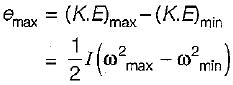QUESTION: 4

The maximum fluctuation of energy during a cycle for a flywheel is

Solution: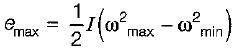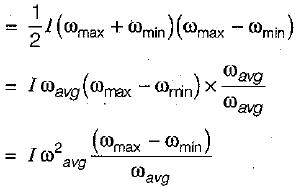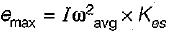QUESTION: 5

The amount of energy absorbed by a flywheel is determined from the

Solution:

The amount of energy absorbed by a flywheel is determined form the torque-crank angle diagram. The area under torque-crank angle diagram is known as turning moment.

QUESTION: 6

Why is the mass of flywheel concentrated in the rim?

Solution:

Flywheel stores energy in the form of inertia and its interia will be more for heavy mass at its periphery. This is the reason mass of flywheel is concentrated in the rim.

QUESTION: 7

The safe rim velocity of a flywheel is influenced by the

Solution:

Rim velocity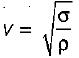where
σ = centrifugal stress of circumferential stress
ρ = density of rim materia!
Hence, rim velocity is the function of centrifugal stress and density of rim material.

QUESTION: 8

The crank-effort diagram of an engine running a machine is showing the areas above and below the mean line (in joules). What is the maximum fluctuation of energy in the below diagram?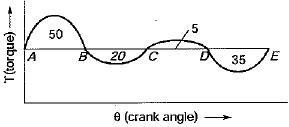Solution: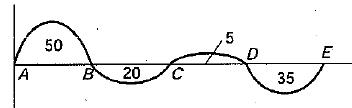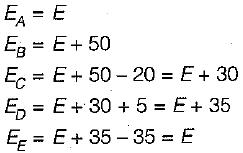Maximum energy = E + 50
Minimum energy = E
Maximum fluctuation of energy
= E + 50 - E = 50J

QUESTION: 9

For a certain engine having an average speed of 1200 rpm, a flywheel approximated as a solid disc, is required for keeping the fluctuation of speed within 2% about the average speed. The fluctuation of kinetic energy per cycle is found to be 2 kJ. What is the least possible mass of the flywheel if its diameter is not to exceed 1 m?

Solution:

Average speed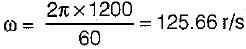we know that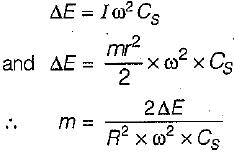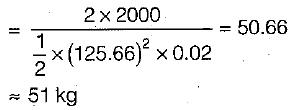QUESTION: 10

Which of the following statement is correct?

Solution:

Flywheel is used to control the variations in speed during each cycle of an engine. While the function of a governor is to maintain the speed of an engine within specified limit whenever, there is a variation of load.